# Catch up on the latest AI articles# Can We Predict Diseases From Unknown Ecological Substances MiRNAs? A Proposal For A Disease Prediction Model Using Reinforcement Learning

3 main points
✔️ Aim to develop a simulator to predict the association between unknown miRNAs and in disease
✔️ Combine three sub-models based on Q-learning algorithm - CMF, NRLMF, and LapRLS - and optimize them for optimal weights
✔️ Case studies of three diseases identified all top 50 miRNAs associated with colorectal and breast neoplasms

written by Jianping Chen
(Submitted on 5 Dec 2021)
Biomolecules

The images used in this article are from the paper, the introductory slides, or were created based on them.

## background

### Is it possible to predict the association between unknown miRNAs and disease?

In this study, we propose a system to simulate the relationship between miRNAs and diseases, which is attracting attention as a new liquid biopsy - a diagnostic technique using minimally invasive specimens such as plasma and urine. miRNAs are biological substances that are highly associated with various diseases including cancer. However, due to the novelty of the field, there are many unanswered questions, and biological experiments are the mainstream method of analysis, which poses challenges in implementation, such as small scale and large investment in manpower and material resources. Therefore, it is important to reduce the cost of experiments. Therefore, to reduce the cost of experiments, there is a need for simulations that point in the direction of the relationship between unknown miRNAs and diseases. In this study, we propose a simulation model based on reinforcement learning Q-learning and combining three sub-models - CMF, NRLMF, and LapRLS - called RFLMDA -is proposed to predict the association between unknown miRNAs and diseases with high accuracy.

### What is miRNA?

First of all, I will briefly explain miRNAs, which are the subject of analysis in this study.

miRNAs are 21-25 nucleotide (nt) long single-stranded RNA molecules, which are biological substances involved in the regulation of post-transcriptional expression of genes in eukaryotes. It is thought that more than 1000 miRNAs are encoded in the human genome. miRNAs destabilize target mRNAs - messenger RNAs - and suppress protein production through translational repression. The proteins that make up the human body are generally converted from DNA to protein by central dogma - the process of converting DNA to mRNA: transcription, and the process of converting mRNA to protein: translation. miRNAs are thought to be the most important regulators of the latter process and are associated with many biological phenomena, including the expression of disease. In particular, it has been reported to be specifically expressed in certain cancer types and stages, and to be highly relevant in the identification of many diseases and viral infections. Therefore, miRNAs can be utilized as novel biomarkers for disease diagnosis and prognosis, and are also expected to have the potential for gene therapy using miRNAs.

On the other hand, since miRNAs are a relatively newly discovered field, there are many unanswered questions, and biological experiments are the mainstream for analysis; therefore, the challenges of such analysis - small scale, large investment in manpower and material resources, long experimental period, the existence of limitations -are present. Against this backdrop, there is a need to develop simulators that point in a certain direction for the association between miRNAs and diseases, thereby reducing the challenges and costs of biological experiments.

## purpose of one's research

In this study, we propose a method to simulate the association between miRNAs and diseases - RFLMDA - by using three models and reinforcement learning to solve these problems - reducing the experimental cost. Specifically, we combine three sub-models - CMF, NRLMF, and LapRLS - and assign optimal weights S based on the Q-learning algorithm of reinforcement learning. The evaluation results for these proposed models - RFLMDA - show that it achieves high prediction accuracy: in comparison with other methods, it is clear that RFLMDA has better prediction accuracy - AUC, AUPR -); simulation results for eight diseases report the derivation of many of the top 50 miRNAs associated with these diseases.

## technique

### data set

The dataset was obtained from a database of miRNA-disease associations in humans - HMDD v2.0 (http://www.cuilab.cn/hmdd, accessed date on 15 October 2021) data (see table below).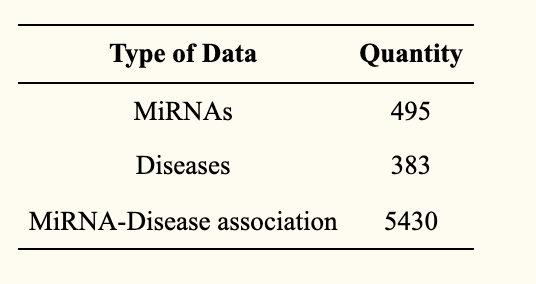It is also assumed that there are interactions between miRNAs and that these interactions influence biological processes. Therefore, in this study, we constructed a 495-row, 495-column miRNA functional similarity adjacency matrix (below) to train for these effects: in the matrix, each element of the matrix refers to the functional similarity score between two miRNAs.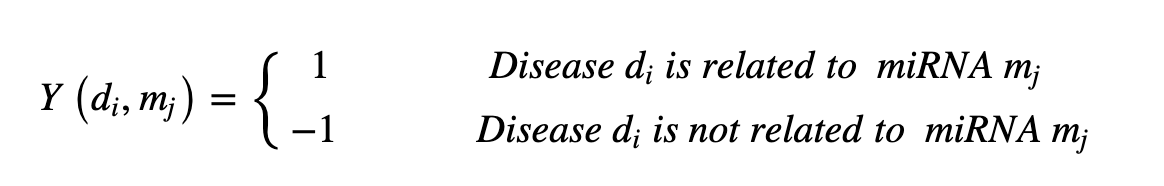We also define semantic similarity for diseases, as they are expected to have similarities with each other (see below): each disease is based on a directed acyclic graph (DAG) - a directed graph where dots represent diseases and edges represent relationships between diseases. The following equation is used.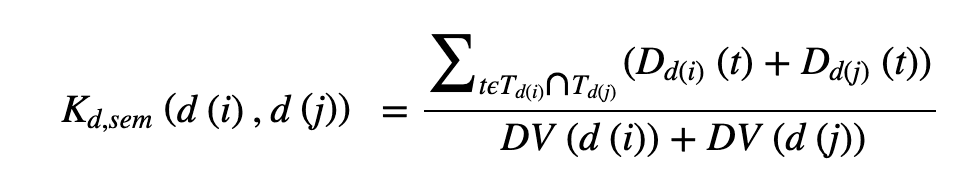### submodel

We will now discuss the three sub-models - CMF, NRLMF, and LapRLS - on which the proposed method is based. Collaborative Matrix Factorization (CMF) is one of the classical models used in recommendation systems, such as rating prediction or cold-start recommendation: it works by minimizing the objective function A: miRNA feature matrix, B: disease feature matrix, F: matrix of predicted miRNA-disease interactions.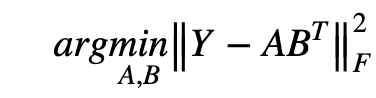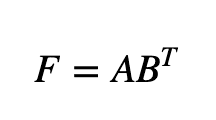Next, we discuss Neighborhood Regularized Logistic Matrix Factorization (NRLMF). This model combines the logistic matrix factorization (LMF) method with regularization to predict the association. To minimize the objective function (below), the model calculates the derivative concerning specific variables - U and V in the equation below - and optimizes based on the extreme values.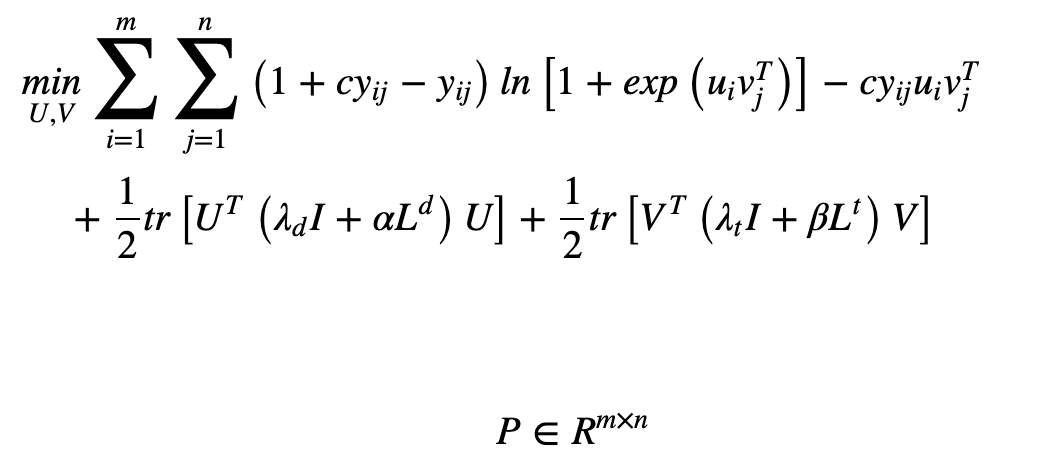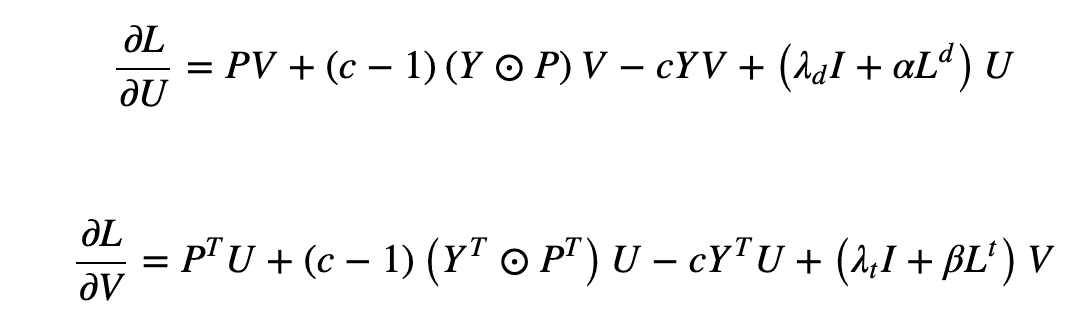The last one is Laplacian Regularized Least Squares (LapRLS), which is a kind of semi-supervised learning method. This model constructs a nearest neighbor graph and makes use of regularization - introducing a Laplacian graph for the coefficients of the least-squares loss function (see below). It also has two main features: the simplicity of the model; performance comparable to Laplacian regularization support vector machines.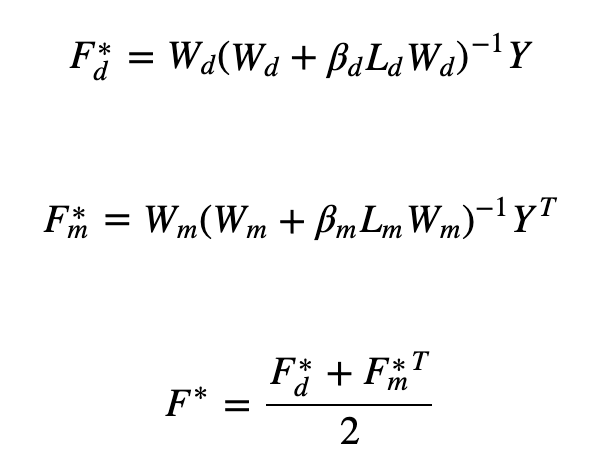## proposed model

The proposed model exploits Q-learning, a type of reinforcement learning algorithm, and consists of assigning optimal weights to the above three models - CMF, NRLMF, and LapRLS. Reinforcement learning is based on four main components: agents, rewards, environmental states, and actions: agents manipulate their environment by taking actions and moving from one state to the next; after completing a task, agents are given a positive or negative When the task is completed, the agent is given a positive or negative reward. One of the characteristics of reinforcement learning is that it derives the best solution for a given situation by selecting actions to maximize the cumulative reward.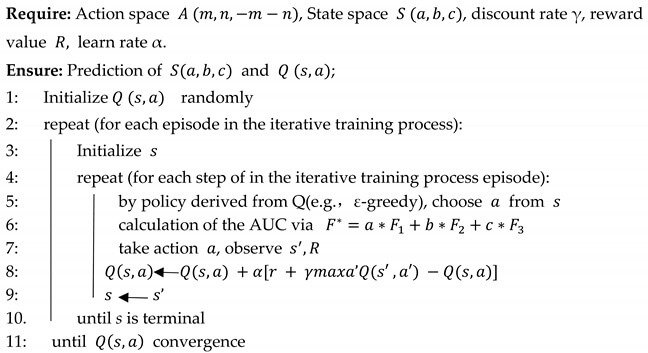Also frequently used in such reinforcement learning is Q-leaning - a Value-based learning algorithm that solves for the Bellman equation based on a Markov model and uses a time difference method for off-policy learning (see figure below).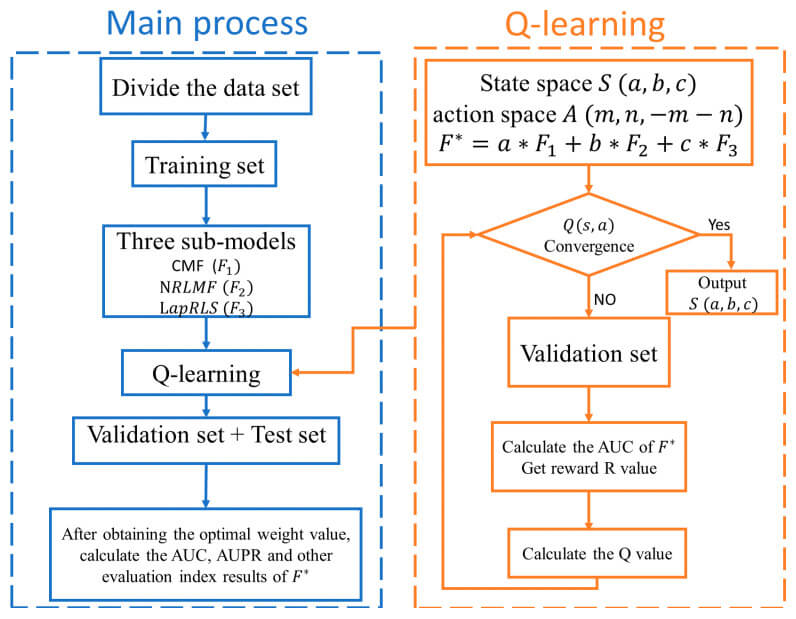Based on these methods, the proposed model derives the optimal weights and assigns them to each model: 5 fold cross-validation - dividing the dataset into training, validation, and test sets in a ratio of 8:1:1 - and The optimal weights are derived and combined.

We use the difference in AUCs as a measure of action selection in reinforcement learning - a new AUC is generated in each round of validation, and the difference in AUCs is used as a measure of reward: if the difference in AUCs between the next and current state is greater than 0, we give a positive 1 reward if the difference in AUC between the next and current state is greater than 0; otherwise, a minus 1 reward is given. These successive iterations of learning allow the rewards to continuously converge and approach the optimal solution. The specific algorithm of the proposed model is as follows.

## result

The purpose of this evaluation is to derive the optimal weights for the proposed model and to derive the prediction accuracy for the association between miRNAs and diseases.

### valuation index

As evaluation indices, we use AUC (Area Under Curve) - the area of the plane graph surrounded by the ROC curve and the horizontal axis, with a range of values from 0 to 1 - and AUPR (area under the PR curve) - the area surrounded by the PR curve ( precision-recall curve): the area surrounded by Recall on the X-axis and Precision on the Y-axis.

### Comparison with existing models

The purpose of this evaluation was to derive the predictive performance of the proposed model, RFLMDA. Specifically, five models including the proposed model - RFLMDA, Mean weighted: three sub-models with 1/3 weights, CMF, NRLMF, LapRLS - are compared and the above evaluation metrics - AUC, AUPR AUPR- are derived. The evaluation results (see below) show that the proposed model performs the best.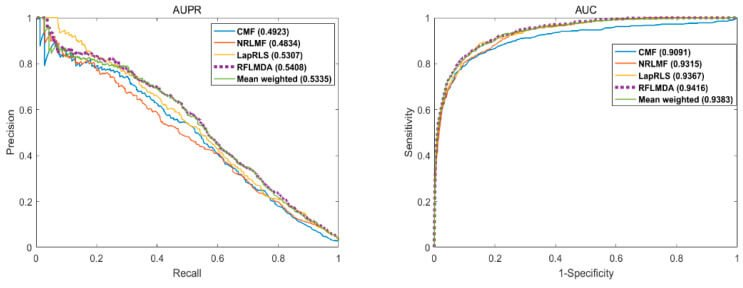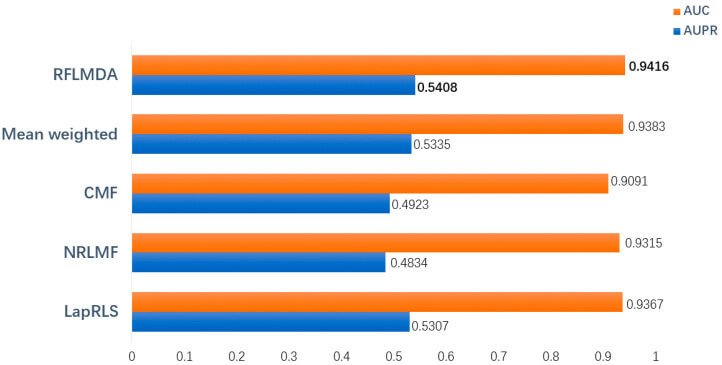### case study

This evaluation utilizes case studies to further clarify the predictive performance of the model RFLMDA - this evaluation allows for a more objective and effective assessment of predictive performance. Here, we investigate the association between miRNAs and diseases reported in HMDD for eight diseases: breast tumors, stomach tumors, colon tumors, pancreatic tumors, esophageal tumors, kidney tumors, lymphomas, and colorectal neoplasms. The evaluation results (table below) show that many of the top 50 most relevant miRNAs are estimated in these diseases.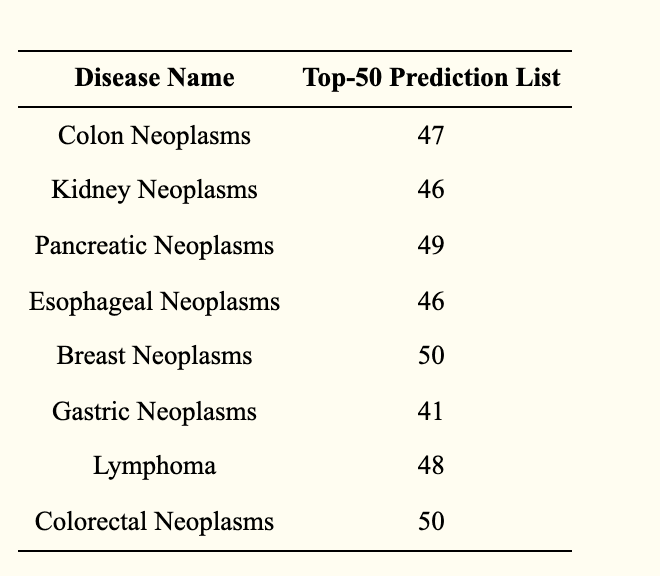## consideration

In this work, we propose a model - RFLMDA - based on the Q-learning algorithm of reinforcement learning and combining three models - CMF, NRLMF, and LapRLS. The model learns the weights: S for the three models to be the optimal values through multiple iterative updates. The results of 5 fold cross-validation evaluation show that the optimal weights are S (0.1735,0.2913,0.5352) and the model has higher prediction accuracy - AUC: 0.9416. In addition, the comparison with other methods shows that the RFLMDA model has a higher performance.

To further validate the predictive performance of RFLMDA, we are conducting additional evaluations as case studies for eight diseases. As a result, all of the top 50 miRNAs related to colorectal neoplasm and breast neoplasm were confirmed, and 41, 47, 49, 46, 46, and 48 of the top 50 miRNAs related to gastric cancer, colorectal cancer, pancreatic cancer, esophageal cancer, kidney cancer, and lymphoma, respectively, were confirmed. These evaluation results indicate that RFLMDA can derive highly reliable candidates in miRNAs.

As the concept of miRNA itself is new, there is a high need for a tool that can simulate the relationship between unknown miRNAs and diseases. Therefore, the proposed model can provide a direction for the degree of association between unknown miRNAs and diseases, which will lead to more effective progress in the development of human disease treatment and genetic medicines.

On the other hand, there are two issues: one is that the performance of the proposed model is limited by the models used, and the other is that the performance of the proposed model is unclear for diseases other than those to be evaluated. In the former case, the prediction accuracy of the proposed model may vary depending on the performance of the three models employed in this study - CMF, NRLMF, and LapRLS; to address this issue, we will consider testing a model that combines other learning and classification models. models. The latter would require additional evaluation of non-case study diseases, especially lifestyle-related diseases that are in high demand for solutions: diabetes, cardiovascular disease, etc. Due to the unique characteristics of each disease, additional algorithms other than Q-learning - model-based learning: Dynamic Programming (DP), other model-free learning: SARSA, Monte Carlo, etc., and redefinition of the similarity matrix defined here In this paper, we will discuss how to redefine the similarity matrix defined here.

If you have any suggestions for improvement of the content of the article,